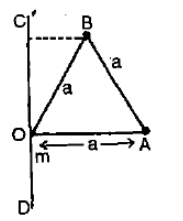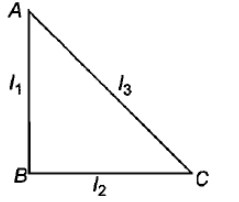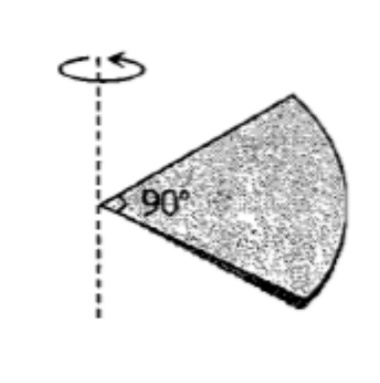Three-point masses 'm' each, are placed at the vertices of an equilateral triangle of side a. Moment of inertia of the system through a mass m at O and lying in the plane of COA & perpendicular to OA is1. $2m{a}^{2}$

2. $\frac{2}{3}m{a}^{2}$

3. $\frac{5}{4}m{a}^{2}$

4. $\frac{7}{4}m{a}^{2}$

Concept Questions :-

Moment of inertia
High Yielding Test Series + Question Bank - NEET 2020

Difficulty Level:

A particle is moving in a circular orbit with constant speed. Select wrong alternate

1. Its linear momentum is conserved

2. Its angular momentum is conserved

3. It is moving with variable velocity

4. It is moving with variable acceleration

Concept Questions :-

Linear momentum
High Yielding Test Series + Question Bank - NEET 2020

Difficulty Level:

ABC is a right angled triangular plate of uniform thickness. The sides are such that AB > BC as shown in figure.  and ${\mathrm{I}}_{3}$ are moments of inertia about AB, BC and AC respectively. Then, which of the following relation is correct?Concept Questions :-

Moment of inertia
High Yielding Test Series + Question Bank - NEET 2020

Difficulty Level:

One solid sphere A and another hollow sphere B are of same mass and same outer radii. Their moment of inertia about their diameters are respectively IA and IB such that

1. ${\mathrm{I}}_{\mathrm{A}}={\mathrm{I}}_{\mathrm{B}}$

2. ${\mathrm{I}}_{\mathrm{A}}>{\mathrm{I}}_{\mathrm{B}}$

3. ${\mathrm{I}}_{\mathrm{A}}<{\mathrm{I}}_{\mathrm{B}}$

4. $\frac{{\mathrm{I}}_{\mathrm{A}}}{{\mathrm{I}}_{\mathrm{B}}}=\frac{{d}_{A}}{{d}_{B}}$

Concept Questions :-

Moment of inertia
High Yielding Test Series + Question Bank - NEET 2020

Difficulty Level:

A couple produces:                                                               [NTSE 1995; CBSE PMT 1997; DCE 2004]

(1) Purely linear motion

(2) Purely rotational motion

(3) Linear and rotational motion

(4) No motion

High Yielding Test Series + Question Bank - NEET 2020

Difficulty Level:

A particle of mass 1 kg is kept at (1m, 1m, 1m). The moment of inertia of this particle about z-axis would be

1.

2.

3.

4.  None of these

High Yielding Test Series + Question Bank - NEET 2020

Difficulty Level:

One quarter sector is cut from a uniform circular disc of radius R.  This sector has mass M.  It is made to rotate about a line perpendicular to its plane and passing through the centre of the original disc.  Its moment of inertia about the axis of rotation is [IIT-JEE (Screening) 2001](1) $\frac{1}{2}M{R}^{2}$

(2) $\frac{1}{4}M{R}^{2}$

(3) $\frac{1}{8}M{R}^{2}$

(4) $\sqrt{2}M{R}^{2}$

High Yielding Test Series + Question Bank - NEET 2020

Difficulty Level:

A wheel is rotating at the rate of 33 rev/min.  If it comes to stop in 20 s. Then, the angular retardation will be

(1) $\mathrm{\pi }\frac{\mathrm{rad}}{{\mathrm{s}}^{2}}$                          (2)

(3)               (4)

High Yielding Test Series + Question Bank - NEET 2020

Difficulty Level:

A solid sphere is rotating about a diameter at an angular velocity $\omega$.  If it cools so that its radius reduces to $\frac{1}{n}$ of its  original value, its angular velocity becomes [MP PMT 2006]

(1) $\frac{\omega }{n}$                                (2) $\frac{\omega }{{n}^{2}}$

(3) $n\omega$                                (4) ${n}^{2}\omega$

High Yielding Test Series + Question Bank - NEET 2020

Difficulty Level:

A horizontal platform is rotating with uniform angular velocity around the vertical axis passing through its centre. At some instant of time a viscous fluid of mass 'm' is dropped at the centre and is allowed to spread out and finally fall. The angular velocity during this period

1. Decreases continuously

2. Decreases initially and increases again

3. Remains unaltered

4. Increases continuously

Concept Questions :-

Angular momentum